# Solutions of Reflection of Light (Page No- 209) - Physics By Lakhmir Singh, Class 10 Class 10 Notes | EduRev

## Class 10 : Solutions of Reflection of Light (Page No- 209) - Physics By Lakhmir Singh, Class 10 Class 10 Notes | EduRev

The document Solutions of Reflection of Light (Page No- 209) - Physics By Lakhmir Singh, Class 10 Class 10 Notes | EduRev is a part of the Class 10 Course Class 10 Physics Solutions By Lakhmir Singh & Manjit Kaur.
All you need of Class 10 at this link: Class 10

Lakhmir Singh Physics Class 10 Solutions Page No:209

Question 1: An object is kept at a distance of 5 cm in front of a convex mirror of focal length 10 cm. Calculate the position and magnification of the image and state its nature.

Solution :

u = -5cm f -10cm

We know that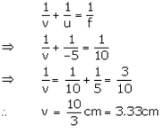The position of the image is 3.33 cm behind the convex mirror. Magnification. m= -v/u = -3.33/-5 = 0.66 The image is virtual and erect.

Question 2: An object is placed at a distance of 10 cm from a convex mirror of focal length 5 cm.
Draw a ray-diagram showing the formation of image.
State two characteristics of the image formed.
Calculate the distance of the image from mirror.

Solution :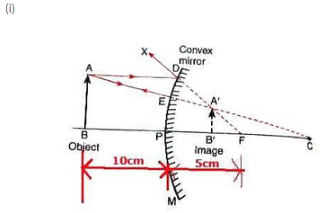(ii) The image formed is diminished and erect.
(iii) u=-10cm. f = 5cm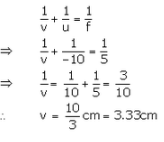Question 3: An object is placed at a distance of 6 cm from a convex mirror of focal length 12 cm. Find the position and nature of the image.

Solution : u= -6cm f = 12cm. v = ?

We know that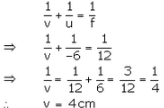Image will be formed 4 cm behind the mirror.

Since the image is formed behind the convex mirror, it is virtual and erect.

Question 4: An object placed 20 cm in front of a mirror is found to have an image 15 cm (a) in front of it, (b) behind the mirror. Find the focal length of the mirror and the kind of mirror in each case.

Solution : (a) u =-20cm, v = -15cm

We know that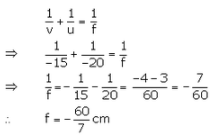The mirror is a concave mirror.
(b) u = -20cm v = 15cm

We know that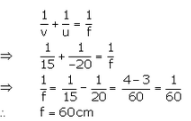The mirror is a convex mirror.

Question 5: An arrow 2.5 cm high is placed at a distance of 25 cm from a diverging mirror of focal length 20 cm. Find the nature, position and size of the image formed.

Solution : h= 2.5cm.u = -25cm. f = 20cm

We know that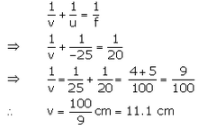The image is formed 11.1cm behind the convex mirror.

Now.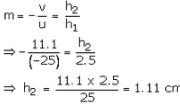The image is virtual. erect and 1.11cm tall.

Question 6: A convex mirror used as a rear-view mirror in a car has a radius of curvature of 3 m. If a bus is located at a distance of 5 m from this mirror, find the position of image. What is the nature of the image ?

Solution : Ra = 3 m  u = -5m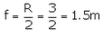We knoe that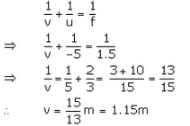The image is formed 1.15 m behind the mirror.

The image is virtual and erect.

Question 7: A diverging mirror of radius of curvature 40 cm forms an image which is half the height of the object. Find the object and image positions.

Solution : R = 40cm

f = R/2 = 10/2 = 20tm

Image is half the height of the object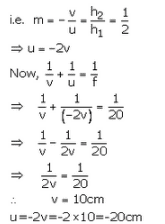So. the object is placed 20 cm in front of the mirror and the image is formed 10cm behind the mirror.

Question 8: The radius of curvature of a convex mirror used as a rear view mirror in a moving car is 2.0 m. A truck is coming from behind it at a distance of 3.5 m. Calculate (a) position, and (b) size, of the image relative to the size of the truck. What will be the nature of the image ?

Solution :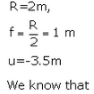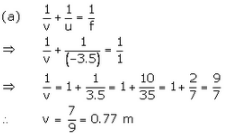So, the image is formed 0.77m behind the mirror.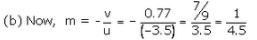As m is positive, so image formed is virtual and erect

Question 9: (a) Draw a diagram to represent a convex mirror. On this diagram mark principal axis, principal focus F
and the centre of curvature C if the focal length of convex mirror is 3 cm.
(b) An object 1 cm tall is placed 30 cm in front of a convex mirror of focal length 20 cm. Find the size and position of the image formed by the convex mirror.

Solution :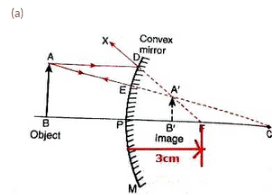(b) h1 = 1cm. u = -30cm f = 20cm. h2= ?, v = ?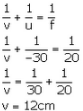The image is formed 12 cm behind the mirror.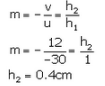Question 10: A shop security mirror 5.0 m from certain items displayed in the shop produces one-tenth magnification.
(a) What is the type of mirror ?
(b) What is the radius of curvature of the mirror ?

Solution :

(a) The mirror is of convex type.
(b) u = -5cm. m = 1/10

We have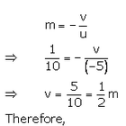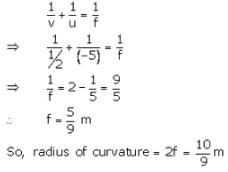Offer running on EduRev: Apply code STAYHOME200 to get INR 200 off on our premium plan EduRev Infinity!

94 docs

,

,

,

,

,

,

,

,

,

,

,

,

,

,

,

,

,

,

,

,

,

,

,

,

;